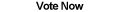Home Articles Components Code Snippets Links# C# Bucket Sort

## Bucket Sort

Sorting in programming is a huge topic, not just in C#. One of the simplest sorting algorithms is the famous bucket sort.

Bucket sorting in C# consists of taking an array of elements that have some sort of numeric value. Each element is stored in a "bucket", using the value as an index. When the bucket is emptied, the result will be a sorted list in order. The space requirements for n elements is n and the running time can be characterized as O(n) since elements are directly being stored in a bucket.

## Integer Example

The simplest example is to sort integers with the bucket sort algorithm. Integers are elements that are values as well. So say you have an array of integers: 3, 67, 5, 23, 5

Since you want a bucket array that can directly add elements, for the above example, the bucket would need to be size 67 (the largest element). However to save space, you can subtract every element by 3 (the smallest element) when adding them to the bucket. In this example we would save 3 cell spaces, but imagine your smallest element is 57, you would save 57 spaces.

Another point to notice is that there can be duplicate elements. Which means the bucket cannot directly store just int values because there'd be no way to tell exactly how many of each value there is. The solution is to make the bucket an array of List<> items. That way elements are added to a list at index i.

Also note the fact that storing equal elements in a list makes the bucket sort algorithm a stable sort, meaning that equal elements stay in the same order. It might not be important with integers, but it's important with other types of objects.

## Bucket Sort Steps

The simple steps laid out in a list come out to:

• Find the maximum and minimum values in the array
• Initialize a bucket array of List<> elements (size is maxValue - minValue + 1)
• Move elements in array to the bucket
• Write the bucket out (in order) to the original array

The nice thing about the bucket sort algorithm is that it is very simple to code.

## Performance Analysis

You might have noticed that the bucket sort does not run as fast as Microsoft's built-in Array.Sort. This is strange at first because Array.Sort uses QuickSort which has a running time of O(n log n) as opposed to the bucket sort's O(n). Unfortunately it makes sense because there are additional factors to consider. For example bucket sort has to use a lot more memory than an in-place QuickSort has to use.

However it is good to know how to use bucket sort because it is the basis for sorting algorithms like radix sort which can be a lot faster with specialized types of data.

Now that you know what bucket sort is all about, find out how to write a faster bucket sort algorithm.

Back to C# Article List